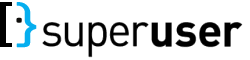select sum(Up) as UpSum, sum(Down) as DownSum from ( select

0

(click on this box to dismiss)Q&A for computer enthusiasts and power users

select
sum(Up) as UpSum,
sum(Down) as DownSum
from (
select
(select count(*) from Votes v where v.VoteTypeId = 2 and v.PostId = p.Id) as Up,
(select count(*) from Votes v where v.VoteTypeId = 3 and v.PostId = p.Id) as DownHold tight while we fetch your results